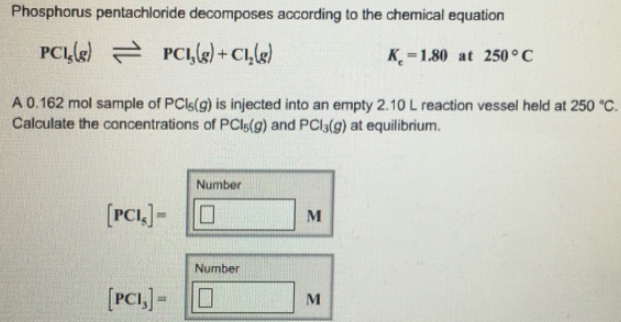# Problem: Phosphorus pentachloride decomposes according to the chemical equation PCl5 (g) ⇌ PCl3 (g) + Cl2(g)                 K c = 1.80 at 250 °C.A 0.162 mol sample of PCl5 (g) is injected into an empty 2.10 L reaction vessel held at 250 °C. Calculate the concentrations of PCl5 (g) and PCl3 (g) at equilibrium.

###### FREE Expert Solution
87% (456 ratings)###### Problem Details

Phosphorus pentachloride decomposes according to the chemical equation

PCl5 (g) ⇌ PCl3 (g) + Cl2(g)                 K c = 1.80 at 250 °C.

A 0.162 mol sample of PCl5 (g) is injected into an empty 2.10 L reaction vessel held at 250 °C. Calculate the concentrations of PCl5 (g) and PCl3 (g) at equilibrium.